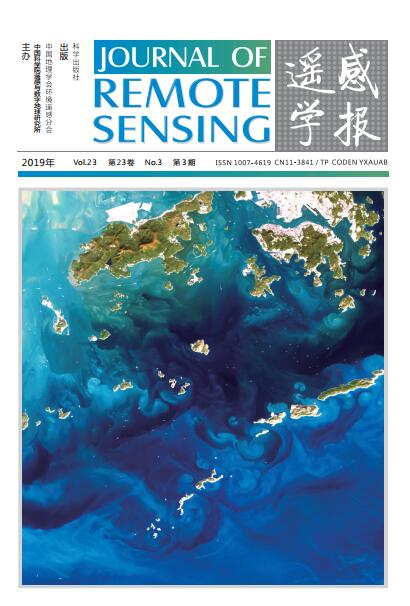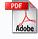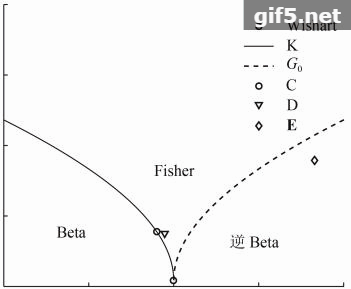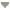出版日期: 2019-05-25 点击次数： 下载次数： DOI: 10.11834/jrs.201974312019 | Volumn23 | Number 3上一篇  |  下一篇G0分布下基于白化滤波的极化SAR图像CFAR检测张嘉峰1,2 , 张鹏1 , 王明春1 , 刘涛1
1. 海军工程大学 电子工程学院，武汉 430030
2. 中国人民解放军 92118部队，舟山 316000
 收稿日期: 2018-07-12; 预印本: 2018-08-24 基金项目: 国家自然科学基金(编号：61372165，61771483) 第一作者简介: 张嘉峰，1993年生，男，助理工程师，研究方向为雷达极化信息处理、新体制雷达技术及电子战建模与仿真。E-mail：751971863@qq.com

# 关键词

CFAR detection method of polarimetric SAR imagery based on whitening filter under G0 distributionZHANG Jiafeng1,2 , ZHANG Peng1 , WANG Mingchun1 , LIU Tao1
1.School of Electronic Engineering, Naval University of Engineering, Wuhan 430030, China
2.No.92118 Unit of PLA, Zhoushan 316000, China

# Abstract

Poor applicability has been a common issue among high-resolution clutter models that use the existing constant false alarm rate (CFAR) detection methods for polarimetric synthetic aperture radar (PolSAR) imageries. To solve the problem, a CFAR detection method with G0 distribution and closed analytical expression for PolSAR imageries is proposed. CFAR loss (CL) is used to quantitatively evaluate the maintenance performance of CFAR detection methods. First, the probability density function (PDF) of the multi-look polarization whitening filter (MPWF) metric is derived on the basis of product modeling by combining the hypotheses of inverse Gamma distribution texture. Second, the PDF of the MPWF metric is integrated, and the analytical expression of the false alarm rate with respect to the CFAR detection threshold is obtained. The process of the proposed CFAR detection method is also designed. Finally, the simulation data and the AIRSAR real data are used to compare the running times of the methods, the fitting performances of the detection metrics, and the target detection performances of the existing and proposed methods. Results show that the running time of the proposed method is 3—30 times shorter than those of the existing methods, and the real-time performance of the proposed method is also enhanced. The analytical results of the AIRSAR real data of Tamano (Japan) show that G0 distribution has a good fitting performance with high-resolution non-uniform sea areas. The proposed method can detect targets effectively in both G0 and non-G0 distribution sea areas, and its robustness is better than those of the existing methods. Moreover, the figure of merit of the proposed method is 15.78% higher than those of the other detection methods. The analytical results of CL indicate that the proposed method has good CFAR maintenance performance. Moreover, the closer the clutter log-cumulant scatter points are to the G0 distribution curve, the better the CFAR maintenance performance of the proposed method.

# Key words

polarimetric synthetic aperture radar (PolSAR), constant false alarm rate (CFAR), product model, G0 distribution, multi-look polarization whitening filter (MPWF), false alarm rate, CFAR Loss (CL), figure of merit (FoM)

# 2 MPWF检测量统计建模

 ${s} = \sqrt \tau {y}$ (1)

 ${C} = \frac{1}{L}\sum\limits_{i = 1}^L {{{s}_i}{s}_i^{\rm{H}}} = \frac{1}{L}\sum\limits_{i = 1}^L {{\tau _i}{{y}_i}{y}_i^{\rm{H}}}$ (2)

 ${C} = \tau {Y}$ (3)

 ${Y} = \frac{1}{L}\sum\limits_{i = 1}^L {{y_i}y_i^{\rm{H}}}$ (4)

 ${\textit{z}}= \frac{1}{L}\sum\limits_{i = 1}^L {{s}_i^{\rm{H}}{{\Sigma }^{ - 1}}{{s}_i}} = Tr\left({{{\Sigma }^{ - 1}}{C}} \right)$ (5)

 ${\Sigma } = E\left({C} \right) = E\left(\tau \right)E\left({Y} \right) = E\left(\tau \right){P}$ (6)

 ${\textit{z}} = \frac{\tau }{{E\left(\tau \right)}}Tr\left({{{P}^{ - 1}}{Y}} \right) = {\tau _{\rm{1}}}x$ (7)

 $\gamma \left({d, Ld} \right) = \frac{{{L^{Ld}}{x^{Ld - 1}}}}{{\Gamma \left({Ld} \right)}}{{\rm{e}}^{ - Lx}}$ (8)

 $f\left({y;\alpha, \beta } \right) = \frac{{{\beta ^\alpha }}}{{\Gamma \left(\alpha \right)}}{y^{ - \alpha - 1}}\exp \left({ - \frac{\beta }{y}} \right){\rm{ }}\left({\alpha, {\rm{ }}\beta, {\rm{ }}y > 0} \right)$ (9)

 $\int_0^{ + \infty } {yf\left({y;\alpha, \beta } \right){\rm{d}}y} = 1$ (10)

 $f\left({{\tau _1};{\textit{λ}} } \right) = \frac{{{{\left({{\textit{λ}} - 1} \right)}^{\textit{λ}} }}}{{\Gamma \left({\textit{λ}} \right)}}{\tau _1}^{ - {\textit{λ}} - 1}\exp \left({\frac{{1 - {\textit{λ}}}}{{{\tau _1}}}} \right){\rm{ }}\left({{\textit{λ}}, {\rm{ }}{\tau _1} > 0} \right)$ (11)

 $f\left(z \right) = \int_0^{ + \infty } {\frac{1}{{{\tau _1}}}f\left({x;d, Ld} \right)f\left({{\tau _1};{\textit{λ}} } \right){\rm{d}}{\tau _1}}$ (12)

x=z/τ1代入上式得

 $f\left({\textit{z}} \right) = \frac{{{L^{Ld}}{z^{Ld - 1}}{{\left({{\textit{λ}} - 1} \right)}^{\textit{λ}} }}}{{\Gamma \left({Ld} \right)\Gamma \left({\textit{λ}} \right)}}\int_0^{ + \infty } {\frac{1}{{{\tau _1}^{Ld + {\textit{λ}} + 1}}}\exp \left({\frac{{1 - {\textit{λ}} - Lz}}{{{\tau _1}}}} \right){\rm{d}}{\tau _1}}$ (13)

 $\int_0^{ + \infty } {{x^{s - 1}}\exp \left({ - \alpha {x^{ - h}}} \right){\rm{d}}x = } {h^{ - 1}}{\alpha ^{{s/h}}}\Gamma \left({{{ - s}/h}} \right)$ (14)

 $f\left({\textit{z}} \right) = \frac{{{L^{Ld}}{{\textit{z}}^{Ld - 1}}{{\left({{\textit{λ}} - 1} \right)}^{\textit{λ}} }\Gamma \left({Ld + {\textit{λ}} } \right)}}{{\Gamma \left({Ld} \right)\Gamma \left({\textit{λ}} \right){{\left({{\textit{λ}} + L{\textit{z}} - 1} \right)}^{Ld +{\textit{λ}}}}}}$ (15)

# 3 CFAR检测阈值求解和算法流程

 ${F_{\textit{z}}}\left(T \right) = \int_0^T {\frac{{{L^{Ld}}{{\left({{\textit{λ}} - 1} \right)}^{\textit{λ}} }\Gamma \left({Ld + {\textit{λ}} } \right)}}{{\Gamma \left({Ld} \right)\Gamma \left({\textit{λ}} \right)}}\frac{{{{\textit{z}}^{Ld - 1}}}}{{{{\left({{\textit{λ}} + L{\textit{z}} - 1} \right)}^{Ld +{\textit{λ}}}}}}{\rm{d}}{\textit{z}}}$ (16)

 $\int_0^u {\frac{{{x^{\mu - 1}}{\rm{d}}x}}{{{{\left({1 + \beta x} \right)}^v}}} = \frac{{{u^\mu }}}{\mu }{}_2{F_1}\left({v, \mu ;1 + \mu ; - \beta u} \right)}$ (17)

 $\begin{split} {F_{\textit{z}}}\left(T \right) = & \frac{{{L^{Ld}}\Gamma \left({Ld + {\textit{λ}}} \right)}}{{\Gamma \left({Ld} \right)\Gamma \left({\textit{λ}} \right){{\left({{\textit{λ}} - 1} \right)}^{Ld}}}}\int_0^T {\frac{{{{\textit{z}}^{Ld - 1}}}}{{{{\left({1 + \frac{L}{{{\textit{λ}} - 1}}{\textit{z}}} \right)}^{Ld + {\textit{λ}} }}}}{\rm{d}}z}= \\ & \frac{{\Gamma \left({Ld + {\textit{λ}} } \right)}}{{\Gamma \left({Ld} \right)\Gamma \left({\textit{λ}}\right)Ld}}{\left({\frac{{LT}}{{{\textit{λ}} - 1}}} \right)^{Ld}}{}\\ & {_2}{F_1}\left({Ld + {\textit{λ}}, Ld;1 + Ld; - \frac{{LT}}{{{\textit{λ}} - 1}}} \right) \end{split}$ (18)

 $\begin{split} {P_{fa}} = & 1 - {F_{\textit{z}}}\left(T \right) = \\ & 1 - \frac{{\Gamma \left({Ld + {\textit{λ}} } \right)}}{{\Gamma \left({Ld} \right)\Gamma \left({\textit{λ}} \right)Ld}}{\left({\frac{{LT}}{{{\textit{λ}} - 1}}} \right)^{Ld}}{} \\ & {_2}{F_1}\left({Ld + {\textit{λ}}, Ld;1 + Ld; - \frac{{LT}}{{{\textit{λ}} - 1}}} \right) \end{split}$ (19)

Fig. 1 The process of CFAR detection method

# 4 实验结果及分析

## 4.1 获取阈值的CPU运行时间分析

Table 1 The polarimetric parameters of several typical clutter backgrounds

 σHH ε γ ρ√γ 树林 0.256 0.160 0.890 0.610 草地 0.086 0.190 1.030 0.530 目标 0.980 0.190 1.000 0.280

Fig. 2 The real false alarm probabilities of the two methods

Fig. 3 The comparison of CPU running time with different methods

## 4.2 MPWF检测量拟合性能分析

Fig. 4 Data fitting regions

Fig. 5 The model recognition results of A and B

Fig. 6 The PDF fitting curves of methods

Fig. 7 The false alarm probability fitting curves of methods

## 4.3 CFAR检测结果及分析

### 4.3.1 G0分布区域检测结果分析

CFAR检测区域A、B如图8所示，滤除舰船目标后基于对数累计量进行模型辨识，结果如图9所示。从图7的辨识结果来看，两个区域的k2/k3散点图均落于G0分布曲线上，因此可以判断A、B区域大致服从G0分布。

Fig. 8 The CFAR detection regions

Fig. 9 The model recognition results of A and B

Fig. 10 The detection results of region A

Fig. 11 The detection results of region B

Table 2 The parameters of ships detection results

 检测 区域 等效 视数L 形状 参数λ 恒虚警 概率设置 检测方法 Ngt Ntd Nfa Nom Pd /% FoM /% A 3.48 6.30 10–5 本文方法 种劲松和朱敏慧(2003)方法 张嘉峰等(2018)方法 王娜等(2011)方法 2P-CFAR 7 7 1 0 100.00 87.50 7 7 0 0 100.00 100.00 7 7 5 0 100.00 58.33 7 7 4 0 100.00 63.64 7 7 2 0 100.00 77.78 10–7 本文方法 种劲松和朱敏慧(2003)方法 张嘉峰等(2018)方法 王娜等(2011)方法 2P-CFAR 7 7 0 0 100.00 100.00 7 7 0 0 100.00 100.00 7 7 3 0 100.00 70.00 7 7 1 0 100.00 87.50 7 7 1 0 100.00 87.50 B 3.73 6.30 10–4 本文方法 种劲松和朱敏慧(2003)方法 张嘉峰等(2018)方法 王娜等(2011)方法 2P-CFAR 5 5 0 0 100.00 100.00 5 5 0 0 100.00 100.00 5 5 4 0 100.00 55.56 5 5 3 0 100.00 62.50 5 5 1 0 100.00 83.33 10–6 本文方法 种劲松和朱敏慧(2003)方法 张嘉峰等(2018)方法 王娜等(2011)方法 2P-CFAR 5 5 0 0 100.00 100.00 5 4 0 1 80.00 80.00 5 5 2 0 100.00 71.43 5 5 0 0 100.00 100.00 5 5 0 0 100.00 100.00
 ${\rm{FoM}} = \frac{{{N_{\rm{td}}}}}{{\left({{N_{\rm{fa}}} + {N_{\rm{gt}}}} \right)}}$ (20)

### 4.3.2 非G0分布区域检测结果分析

Fig. 12 The CFAR detection regions

Fig. 13 The model recognition results of C, D and E

Fig. 14 The detection results of region C, D and E

### 4.3.3 恒虚警保持性能分析

 ${C_L} = \left| {20\log \left({{{\bar P}_{\rm{fa}}}/{P_{\rm{fa}}}} \right)} \right|$ (21)

Table 3 Textures of simulation data

 Fisher(A) K(B) G0(C) Wishart(D) Beta(E) 逆Beta(F) α — 3 — — — — λ — — 3 — — — u 5 — — — 2 1 v 6 — — — 2 2 ${\bar{\textit{λ}}}$ 2.5627 1.9774 2.9989 1.5223×103 1.6009 5.8803

A、B、C、D、E、F 6块区域数据的模型辨识结果如图15所示，虚警损失率CL随检测门限T变化如图16所示。由于Wishart分布数据参数估计结果为1.5223×103，数值较大，导致其CL为无穷大，故在图16中无显示。

Fig. 15 The model recognition results of regions

Fig. 16 The relationship between CLand T

# 5 结　论

• Bateman H. 1954. Tables of Integral Transforms. New York: McGraw-Hill Book Company, Inc.: 313
• Boerner W M, Kostinski A and James B. 1988. On the concept of the polarimetric matched filter in high resolution radar imagery: an alternative for speckle reduction//Proceedings of 1988 International Geoscience and Remote Sensing Symposium. Edinburg: [s.n.]: 69–72
• Chong J S and Zhu M H. 2003. Target detection algorithm of SAR image based on local window K-distribution. Journal of Electronics and Information Technology, 25 (9): 1276–1280. ( 种劲松, 朱敏慧. 2003. SAR图像局部窗口K-分布目标检测算法. 电子与信息学报, 25 (9): 1276–1280. )
• Cui H G. 2014. Research on Statistical Modelling and Parameters Estimation of PolSAR Imagery. Wuhan: Naval University of Engineering: 123–125 (崔浩贵. 2014. 极化SAR图像统计建模及其参数估计方法研究. 武汉: 海军工程大学: 123–125)
• Frery A C, Muller H J, Yanasse C C F and Sant’Anna S J S. 1997. A model for extremely heterogeneous clutter. IEEE Transactions on Geoscience and Remote Sensing, 35 (3): 648–659. [DOI: 10.1109/36.581981]
• Goldstein G B. 1973. False-alarm regulation in log-normal and weibull clutter. IEEE Transactions on Aerospace and Electronic Systems, AES-9 (1): 84–92. [DOI: 10.1109/TAES.1973.309705]
• Gradshteyn I S and Ryzhik I M. 2007. Table of Integrals, Series, and Products. 7th ed. San Diego, CA: Academic Press: 316
• He Z G, Zhou X G, Lu J and Kuang G Y. 2009. A fast CFAR detection algorithm based on the G0 distribution for SAR images . Journal of National University of Defense Technology, 31 (1): 47–51. [DOI: 10.3969/j.issn.1001-2486.2009.01.010] ( 贺志国, 周晓光, 陆军, 匡纲要. 2009. 一种基于G0分布的SAR图像快速CFAR检测方法 . 国防科技大学学报, 31 (1): 47–51. [DOI: 10.3969/j.issn.1001-2486.2009.01.010] )
• Jakeman E and Pusey P. 1976. A model for non-Rayleigh sea echo. IEEE Transactions on Antennas and Propagation, 24 (6): 806–814. [DOI: 10.1109/TAP.1976.1141451]
• Jao J. 1984. Amplitude distribution of composite terrain radar clutter and the κ-distribution. IEEE Transactions on Antennas and Propagation, 32 (10): 1049–1062. [DOI: 10.1109/TAP.1984.1143200]
• Khan S and Guida R. 2014. On fractional moments of multilook polarimetric whitening filter for polarimetric SAR data. IEEE Transactions on Geoscience and Remote Sensing, 52 (6): 3502–3512. [DOI: 10.1109/TGRS.2013.2273128]
• Liu G Q, Huang S J, Torre A and Rubertone F. 1998. A new multi-look polarimetric SAR target detector and its performance analysis. Signal Processing, 14 (2): 110–116. ( 刘国庆, 黄顺吉, Torre A, Rubertone F. 1998. 一种新的多视全极化SAR目标检测器及其性能分析. 信号处理, 14 (2): 110–116. )
• Liu G Q, Huang S J, Torre A and Rubertone F. 1998. The multilook polarimetric whitening filter (MPWF) for intensity speckle reduction in polarimetric SAR images. IEEE Transactions on Geoscience and Remote Sensing, 36 (3): 1016–1020. [DOI: 10.1109/36.673694]
• Liu T, Cui H G and Gao J. 2013. Statistics of the determinant of the Wishart distributed matrix and its application to parameter estimation. Acta Electronica Sinica, 41 (6): 1231–1237. [DOI: 10.3969/j.issn.0372-2112.2013.06.030] ( 刘涛, 崔浩贵, 高俊. 2013. Wishart分布矩阵行列式值的统计特性及其在参数估计中的应用. 电子学报, 41 (6): 1231–1237. [DOI: 10.3969/j.issn.0372-2112.2013.06.030] )
• Liu T, Cui H G, Mao T, Xi Z M and Gao J. 2012. Novel estimation of the equivalent number of looks in polari-metric SAR imagery based on log-cumulants of sub-covariance- matrix. Scientia Sinica (Informationis), 42 (11): 1459–1470. [DOI: 10.1360/112011-1189] ( 刘涛, 崔浩贵, 毛滔, 席泽敏, 高俊. 2012. 基于子矩阵对数累积量的极化合成孔径雷达图像等效视图数估计新方法. 中国科学: 信息科学, 42 (11): 1459–1470. [DOI: 10.1360/112011-1189] )
• Liu T, Cui H G, Xi Z M and Gao J. 2016. Novel estimators of equivalent number of looks in polarimetric SAR imagery based on sub-matrices. Science China Information Sciences, 59 (6): 062309 [DOI: 10.1007/s11432-015-5480-x]
• Lopes A and Sery F. 1997. Optimal speckle reduction for the product model in multilook polarimetric SAR imagery and the wishart distribution. IEEE Transactions on Geoscience and Remote Sensing, 35 (3): 632–647. [DOI: 10.1109/36.581979]
• Lu T Z, Zhang J, Ji Y G, Zhang X and Meng J M. 2011. Ship target detection algorithm based on G0 distribution for SAR images under rough sea conditions . Advances in Marine Science, 29 (2): 186–195. [DOI: 10.3969/j.issn.1671-6647.2011.02.008] ( 鲁统臻, 张杰, 纪永刚, 张晰, 孟俊敏. 2011. 基于G0分布的高海况SAR船只目标检测方法 . 海洋科学进展, 29 (2): 186–195. [DOI: 10.3969/j.issn.1671-6647.2011.02.008] )
• Novak L M and Burl M C. 1990. Optimal speckle reduction in polarimetric SAR imagery. IEEE Transactions on Aerospace and Electronic Systems, 26 (2): 293–305. [DOI: 10.1109/7.53442]
• Novak L M, Burl M C and Irving W W. 1991. Optimal polrimetric procesing for enhanced target detetion//Proceedings of 1991 National Telesystems Conference. Atlanta: 69–75
• Novak L M, Sechtin M B, Cardullo M J. 1989. Studies of target detection algorithms that use polarimetric radar data. IEEE Transactions on Aerospace and Electronic Systems, 25 (2): 150–165. [DOI: 10.1109/7.18677]
• Pi Y M, Zou Q and Huang S J. 2002. Speckle reduction of polarimetric SAR—polarimetric whitening filter. Journal of Electronics and Information Technology, 24 (5): 597–603. ( 皮亦鸣, 邹琪, 黄顺吉. 2002. 极化SAR相干斑抑制——极化白化滤波器. 电子与信息学报, 24 (5): 597–603. )
• Stinco P, Greco M and Gini F. 2011. Adaptive detection in compound-Gaussian clutter with inverse-gamma texture//Proceedings of 2011 IEEE CIE International Conference on Radar. Chengdu: IEEE: 434–437 [DOI: 10.1109/CIE-Radar.2011.6159570]
• Wang N, Shi G T, Lu J and Kuang G Y. 2011. A new polarimetric SAR image CFAR target detecting method. Journal of Electronics and Information Technology, 33 (2): 395–400. [DOI: 10.3724/SP.J.1146.2010.00023] ( 王娜, 时公涛, 陆军, 匡纲要. 2011. 一种新的极化SAR图像目标CFAR检测方法. 电子与信息学报, 33 (2): 395–400. [DOI: 10.3724/SP.J.1146.2010.00023] )
• Wang N, Liu L, Hu C B, Kuang G Y and Jiang Y M. 2011. A novel polarimetric CFAR target detection method//Proceedings of 2011 International Workshop on Multi-Platform/multi-Sensor Remote Sensing and Mapping. Xiamen: IEEE: 1–6 [DOI: 10.1109/M2RSM.2011.5697368]
• Wei J J, Li P X, Yang J, Zhang J X and Lang F K. 2014. A new automatic ship detection method using L-band polarimetric SAR imagery. IEEE Journal of Selected Topics in Applied Earth Observations and Remote Sensing, 7 (4): 1383–1393. [DOI: 10.1109/JSTARS.2013.2269996]
• Weinberg G V. 2013. Coherent CFAR detection in compound Gaussian clutter with inverse gamma texture. EURASIP Journal on Advances in Signal Processing, 2013 : 105 [DOI: 10.1186/1687-6180-2013-105]
• Witkovský V. 2001. Computing the distribution of a linear combination of inverted gamma variables. Kybernetika, 37 (1): 79–90.
• Zhang J F, Zhu B, Zhang P, Wang M C and Liu T. 2018. Polarimetric SAR imagery target CFAR detection analytical algorithm with Wishart distribution. Acta Electronica Sinica, 46 (2): 433–439. [DOI: 10.3969/j.issn.0372-2112.2018.02.024] ( 张嘉峰, 朱博, 张鹏, 王明春, 刘涛. 2018. Wishart分布情形下极化SAR图像目标CFAR检测解析方法. 电子学报, 46 (2): 433–439. [DOI: 10.3969/j.issn.0372-2112.2018.02.024] )
• Zhao Y N, Pang X Y and Yin B. 2013. Adaptive radar detection for targets in compound-Gaussian clutter with inverse-gamma texture//Proceedings of IET International Radar Conference 2013. Xi’an: IEEE: 1–4 [DOI: 10.1049/cp.2013.0249]